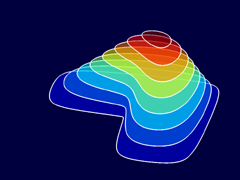Cleve Moler 撰写的教科书

# Numerical Computing with MATLAB

### Book Summary

Numerical Computing with MATLAB is a textbook for an introductory course in numerical methods, MATLAB, and technical computing. It emphasizes the informed use of mathematical software. Topics include matrix computation, interpolation and zero finding, differential equations, random numbers, and Fourier analysis.

Based on MATLAB, the textbook provides more than 70 M-files. Many of the more than 200 exercises involve modifying and extending these programs. The book also makes extensive use of computer graphics, including interactive graphical expositions of numerical algorithms.

Two editions of the book have been published. An electronic edition, published by The MathWorks, is available from this Web site. A traditional textbook print edition, published by the Society for Industrial and Applied Mathematics, is available from the SIAM Web site.

## Companion Curriculum Tools for Instructors

Professors adopting this textbook for a course can get access to curriculum tools and materials, including a solutions manual and a set of slides for use in classroom lectures.### Learn Differential Equations

Up close with Gilbert Strang and Cleve Moler.